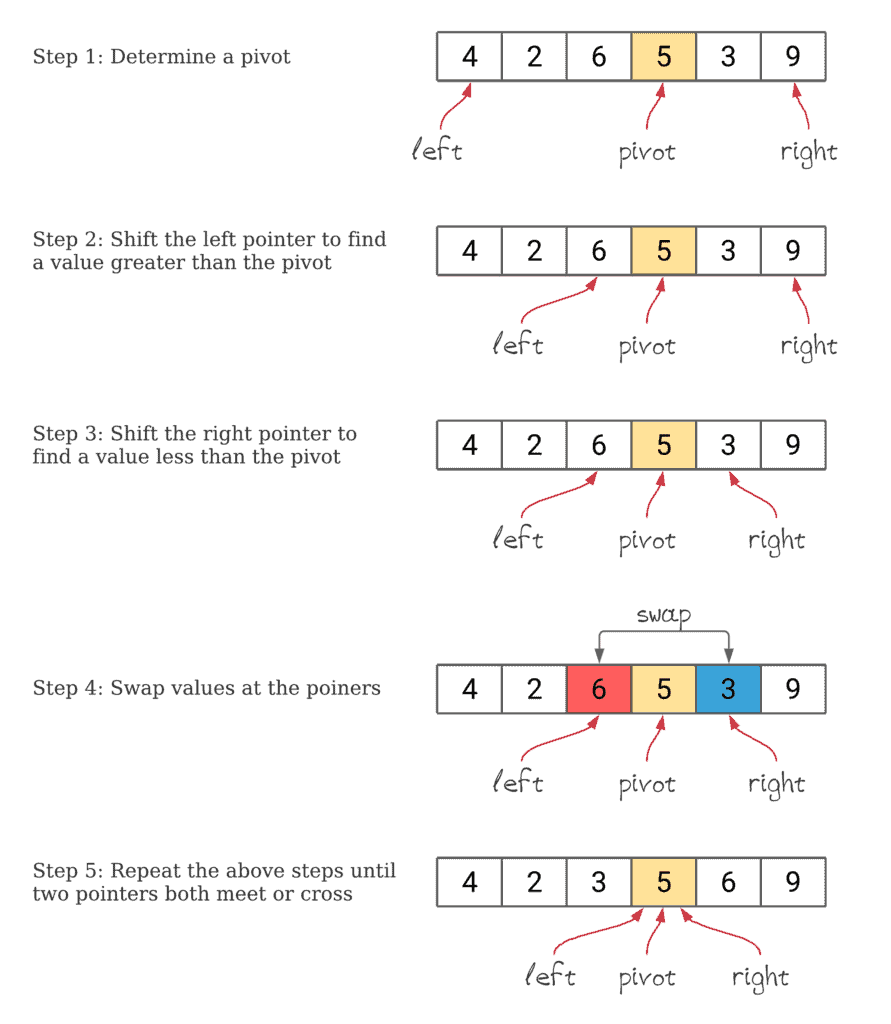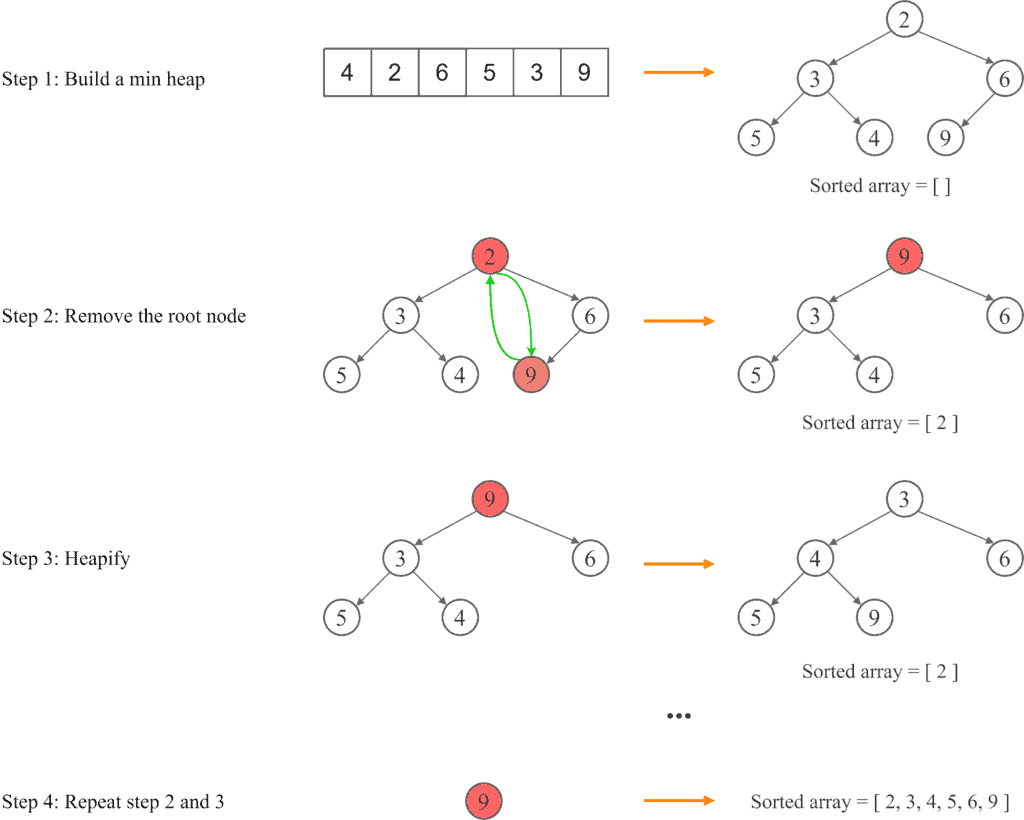## 1. Introduction

In this tutorial, we’ll be comparing two powerful sorting algorithms, namely Quicksort and Heapsort. Quicksort is commonly used in practice because it’s faster, but Heapsort is used when memory usage is a concern.

First, we’ll briefly explain the process of Quicksort and Heapsort algorithms. Then we’ll compare these algorithms, and discuss the advantages and disadvantages of each.

## 2. Quicksort

The Quicksort algorithm is based on the divide-and-conquer approach. Overall, the quicksort algorithm follows three main steps:

• Pick an element from the array as a pivot
• Partition the problem set by moving smaller elements to the left of the pivot and larger elements to its right
• Repeat the above steps on each partition

Let’s take a look at the following illustrations to understand the approach better:In average, as well as best cases, the time complexity of the Quicksort algorithm is. In principle, the worst-case time complexity can beif we select a bad pivot, and it may happen when the array is already sorted in ascending or descending order.
Quicksort is usually implemented as an unstable sort with best-case space complexity ofand average-case space complexity of.

## 3. Heapsort

Heapsort is a comparison-based sorting method based on the binary heap data structure. The binary heap structure allows the Heapsort algorithm to take advantage of the heap’s properties:

• Every node’s value must be greater (or smaller) than all values stored in its children
• It’s a complete tree, which means it has the smallest possible height

We can summarise Heapsort into four main steps:

• Build a min (or max) heap from the input array
• At this point, the smallest item is stored at the root of the heap. We’ll remove the element from the root node, and store the rightmost leaf in the root node.
• Heapify the root of the tree
• Repeat steps 2 and 3 while the size of the heap is greater than 1

Heapify is a process of arranging the nodes in the correct order so that they follow the heap property. For a more in-depth overview of the Heapsort algorithm and an explanation of the heap data structure, we can read these articles which explain Heap Sort in Java and how to Max-Heapify A Binary Tree.

Now let’s apply the concepts of the Heapsort algorithm to the same array we used in our previous example:The time complexity of Heapsort at all cases is, but Heapsort usesauxiliary space, so if memory concerns are an issue, Heapsort might be a good option for a sorting algorithm.

## 4. Quicksort vs. Heapsort

Now that we have an idea of how Quicksort and Heapsort work, let’s compare the basic properties of these two algorithms:The main difference between these two algorithms lies in their method. Heapsort is based on the heap data structure, while Quicksort operates by recursively partitioning the array. The main advantages and disadvantages of each algorithm are:Although Heapsort has the worst-case time complexity of, it’s slower in practice on most machines than a well-implemented Quicksort. This is because Heapsort’shides constants factors that impact the overall performance.

However, Heapsort uses onlyauxiliary space, while Quicksort takes up to, so if memory usage is limited, such as in embedded systems, Heapsort might be a good choice compared to other algorithms.

Quicksort is faster in practice because its inner loop can be efficiently implemented on most architectures. Quicksort can be implemented in different ways by changing the choice of pivot to avoid the worst case.

Furthermore, Quicksort is an internal sorting method where the data is sorted in the main memory, As a result, Quicksort performs better on small datasets rather than on datasets that are too large to fit in the memory.